Courses

# Basic Concept of Rotating Electrical Machines of Electrical Machines - 2

## 15 Questions MCQ Test Topicwise Question Bank for Electrical Engineering | Basic Concept of Rotating Electrical Machines of Electrical Machines - 2

Description
This mock test of Basic Concept of Rotating Electrical Machines of Electrical Machines - 2 for Electrical Engineering (EE) helps you for every Electrical Engineering (EE) entrance exam. This contains 15 Multiple Choice Questions for Electrical Engineering (EE) Basic Concept of Rotating Electrical Machines of Electrical Machines - 2 (mcq) to study with solutions a complete question bank. The solved questions answers in this Basic Concept of Rotating Electrical Machines of Electrical Machines - 2 quiz give you a good mix of easy questions and tough questions. Electrical Engineering (EE) students definitely take this Basic Concept of Rotating Electrical Machines of Electrical Machines - 2 exercise for a better result in the exam. You can find other Basic Concept of Rotating Electrical Machines of Electrical Machines - 2 extra questions, long questions & short questions for Electrical Engineering (EE) on EduRev as well by searching above.
QUESTION: 1

### A 6-pole machine is rotating at a speed of 1200 rpm. This speed in electrical radians per sec and mechanical radian per sec are respectively given by

Solution:

Given,
N = 1200 rpm
∴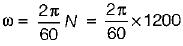= 40π mech.rad/sec
We know that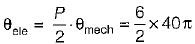= 120π elec-rad/sec

QUESTION: 2

### In an alternator, frequency per revolution is equal to

Solution:

f = NP/120 cycles/sec
or,  frequency/rev = Number of pole pairs

QUESTION: 3

### A three - phase alternator is wound with a 60 degree phase spread armature winding and develops 300 kVA. If the armature is reconnected utilizing all the coils for single-phase operation with a phase spread of 180 degree, the new rating of the machine will be

Solution:

kVA rating, αEf ∝ kd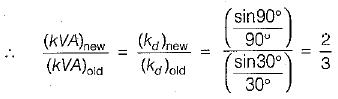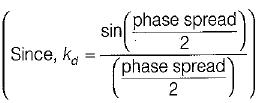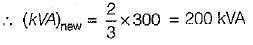QUESTION: 4

A synchronous machine has full-pitch coils having coil-span of 12 slots. For eliminating third harmonic, the coil-span should be

Solution:

To eliminate 3rd harmonic,
3α/2 = 90º
or,
α = 60°
∴ coil span = 180° - 60° = 120°
Now, 180° elec occupy 12 slots.
∴ 120° elec occupy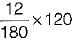= 8 slots
Hence, coil span for eliminating 3rd harmonic = 8 slots.

QUESTION: 5

The 5th harmonic mmf wave, produced by 3-phase currents flowing in 3-phase balanced winding, rotates w.r.t fundamental field (Ns = speed of fundamental mmf wave) at a speed of

Solution:

5th harmonic is a negative sequence quantity which rotates at a speed of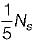w.r.t observer in space while the fundamental field rotates at a speed of Ns in space.
The direction of rotation of 5th harmonic mmf is opposite to that of fundamental mmf wave. Hence, speed of fifth harmonic w.r.t fundamental mmf wave.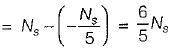QUESTION: 6

Match List- I (Power losses) with List - II (Dependent upon) and select the correct answer using the codes given below the lists:
List-I
A. Stray load loss
B. Brush constant loss
C. Hysteresis loss
D. No-load rotational loss
List-Il
1. Load
2. Value of flux
3. Rotor rotation
4. Square of load
Codes:
A B C D
(a) 3 1 4 2
(b) 4 1 2 3
(c) 3 4 2 1
(d) 4 1 3 2

Solution:

Hysteresis loss,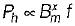Brush constant loss = VDIL  ∝ IL
Stray load loss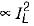No-load rotational loss (friction and windage loss) is due to rotor rotation.

QUESTION: 7

Output rating of
1. dc generators is in kVA.
2. ac motor is in kW.
3. ac generator is in kVA.
4. dc motors is in kW.
5. induction motors is in h.p.
6. synchronous motors is in kVA.
From these, the correct statements are:

Solution:
QUESTION: 8

If Pi be the iron loss and P0 be the ohmic ioss on full load, then which of the following conditions has to be satisfied to obtain maximum efficiency at 2/3 full load?

Solution:

At maximum efficiency, copper loss = iron loss or, x2P0 - Pi (x = fraction of full load)
At 2/3 full-load,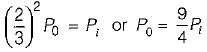QUESTION: 9

If ϕm is the maximum value of flux due to any one phase, the resultant flux in 3-phase and 2-phase ac machines would be given by

Solution:

If n be the number of phases then,
magnitude of resultant flux = n/2 ϕm
(n = 3 for 3-phase and n = 2 for 2-phase)
∴ For 3 phase,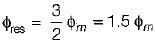and for 2 phase,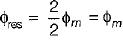Both the resultant flux will be rotating.

QUESTION: 10

Resistance of one conductor for a dc machine is 10 mΩ. What would be the armature circuit resistance if the dc machine with 640 conductors and 8 poles is wave-connected ?

Solution:

For wave-winding, number of conductors per parallel path
= Z/2 = 640/2 = 320
∴ Armature circuit resistance,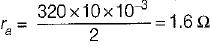QUESTION: 11

A 6-pole, 50 Hz, 3-phase induction motor has a stator bore diameter of 1.2 meters, its stator is cut and laid out flat, so that rotating mmf wave now becomes a travelling mmf wave. What is the linear velocity of the travelling mmf wave in meters per second ?

Solution:

Speed of rotating mmf wave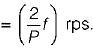As in one revolution, a peripheral distance of πD meter is traversed, therefore speed of travelling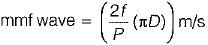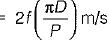= [2f (Poie-pitch)] m/s N
Now, D = 1.2 m , P = 6
∴ Speed of travelling mmf wave is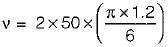= 62.83 m/s

QUESTION: 12

Assertion (A) : In a rotating electrical machine, having 2 poles on the stator and 4 poles on the rotor, the net electromagnetic torque developed is zero.
Reason (R) : In all rotating electrical machines, the number of rotor poles must be equal to the number of stator poles for development of electromagnetic torque.

Solution:
QUESTION: 13

Assertion (A) : Exciting winding is one which produces working flux.
Reason (R) : Armature winding in one in which the working emf is induced by the working flux,

Solution:
QUESTION: 14

Assertion (A) : At the time of winding design of three-phase alternators, the attention is mainly directed for the attenuation of triplen harmonics, by adopting a chording angle of 60° electrical.
Reason (R): Three-phase alternators are invariably star-connected to eliminate triplen harmonics from the line e.m.f.

Solution:

At the time of winding design of 3-phase alternators, the attention is mainly directed for the attenuation of non-triplen harmonics (i.e. 5th and 7th harmonics) because the triplen harmonics (3rd, 9th etc) can be automatically eliminated from line emf if alternator is connected in star.

QUESTION: 15

The induced emf in the armature conductors of a d.c motor is

Solution: